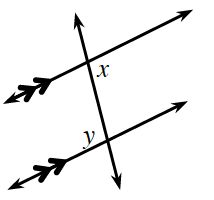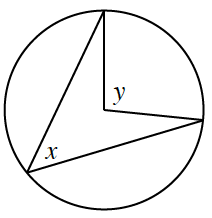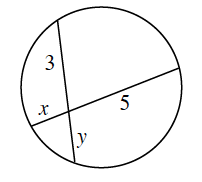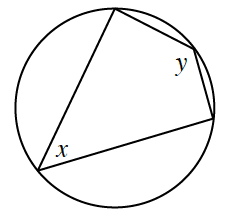### Home > INT2 > Chapter 10 > Lesson 10.2.3 > Problem10-84

10-84.

For each diagram below, write an equation to represent the relationship between $x\text{ and }y$.

1.If two lines cut by transversal are parallel, what are some of the relationships between the angles?

$x = y$

1.What is the relationship between central and inscribed angles?

1.Use the vertical angles created by the chords to form two similar triangles. How can this help you?

$3y = 5x$

1.What is the relationship between an inscribed angle and its intercepted arc? How can this help you?

Recall that the measure of an inscribed angle is half the measure of the arc it intercepts.

$x+y=180°$